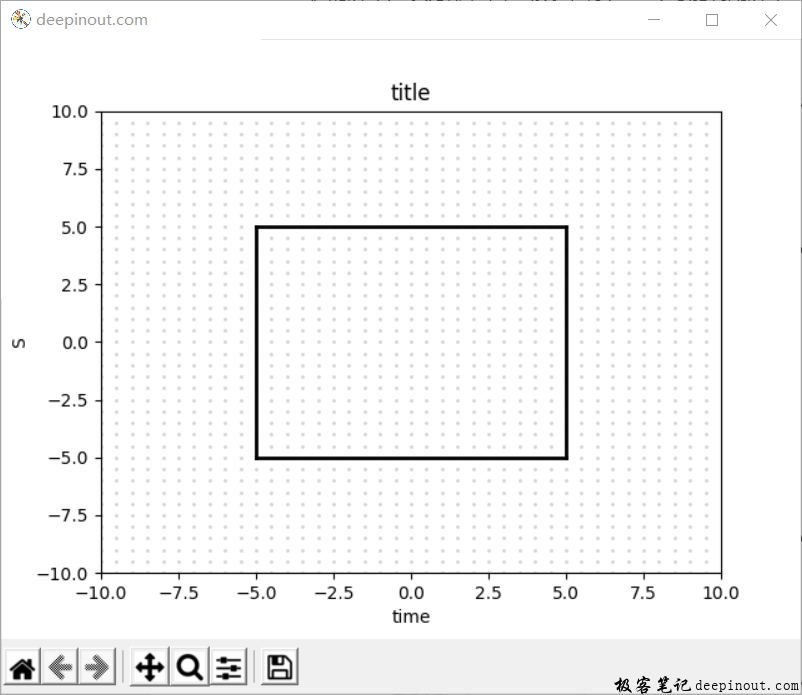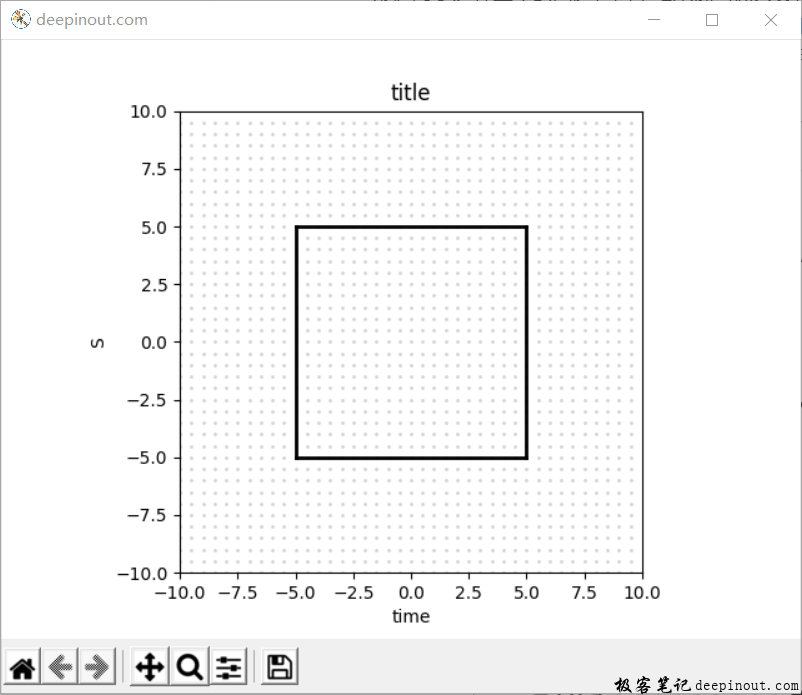# Matplotlib 图形失真

Matplotlib某些方面的应用，需要对画图有一个严格的要求，比如不能把一个长方形显示出来是一个正方形，也不能把一个正方形显示为一个长方形。对于这样显示的问题，简单地来说叫做失真，虽然数值上的坐标点的距离是相等的，但是在屏幕上显示的距离不一样，导致失真。假如老师在教小学生认识正方形，但是老师做出来的课件老是显示为长方形，让学生无法认识正方形。为什么会出现这个问题呢？因为计算机的屏幕是长方形，因此很多程序为了尽可能利用屏幕显示，往往不是数学上严格的比例来显示的，会有一定的比例缩放，这时就会导致失真出现。可以看一下图：import matplotlib.pyplot as plt
import numpy as np

x1, x2 = -10, 10
y1, y2 = -10, 10

fig = plt.figure('deepinout.com')
plt.axis([x1, x2, y1, y2]) #
plt.xlabel('time')
plt.ylabel('S')
plt.title('title')

dx = 0.5
dy = 0.5
for x in np.arange(x1, x2, dx):
for y in np.arange(y1, y2, dy):
plt.scatter(x, y, s = 1, color = 'lightgray')

#画一个正方形
plt.plot([-5, 5], [5, 5], linewidth = 2, color = 'k')
plt.plot([5, 5], [5, -5], linewidth = 2, color = 'k')
plt.plot([5, -5], [-5, -5], linewidth = 2, color = 'k')
plt.plot([-5, -5], [-5, 5], linewidth = 2, color = 'k')

plt.show()


Axes.set_aspect(self, aspect, adjustable=None, anchor=None, share=False)


aspect： 表示坐标轴的显示比例。设置为auto，表示X轴和Y轴坐标显示数据按图的大小来自动设置；设置为equal，表示X轴和Y轴的比例相同；

fig = plt.figure('deepinout.com')
plt.axes().set_aspect('equal')
plt.axis([x1, x2, y1, y2]) #


import matplotlib.pyplot as plt
import numpy as np

x1, x2 = -10, 10
y1, y2 = -10, 10

fig = plt.figure('deepinout.com')
plt.axes().set_aspect('equal')
plt.axis([x1, x2, y1, y2]) #
plt.xlabel('time')
plt.ylabel('S')
plt.title('title')

dx = 0.5
dy = 0.5
for x in np.arange(x1, x2, dx):
for y in np.arange(y1, y2, dy):
plt.scatter(x, y, s = 1, color = 'lightgray')

#画一个正方形
plt.plot([-5, 5], [5, 5], linewidth = 2, color = 'k')
plt.plot([5, 5], [5, -5], linewidth = 2, color = 'k')
plt.plot([5, -5], [-5, -5], linewidth = 2, color = 'k')
plt.plot([-5, -5], [-5, 5], linewidth = 2, color = 'k')

plt.show()• 回顶